#### Unbalanced Assignment Problems, Solved Assignment Problem

Unbalanced Assignment Problems

If the number of columns and rows are not equivalent then such type of problems are known as unbalanced assignment problems.

Example 1

A company has 4 machines on which they need to perform 3 jobs. Each job can be allotted to one and only one machine. The cost of each job on each machine is provided in the following table

 Machines Jobs W X Y Z A 18 24 28 32 B 8 13 17 19 C 10 15 19 22

 18 24 28 32 8 13 17 19 10 15 19 22 0 0 0 0

Row Reduced matrix

 0 6 10 14 0 5 9 11 0 5 9 12 0 0 0 0

I Modified Matrix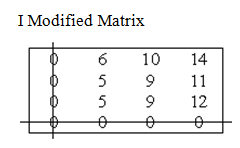N < n i.e. 2 < 4

II Modified Matrix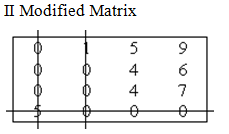N < n i.e. 3 < 4

III Modified Matrix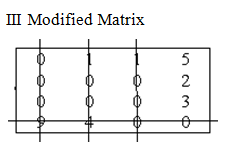N = n

Zero assignment

Multiple assignments presents

Solution Table -I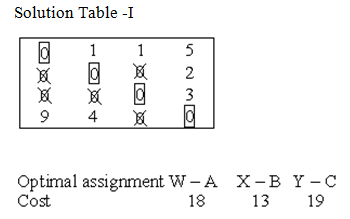Minimum cost = 18 + 13 + 19 = Rs 50

Solution Table -II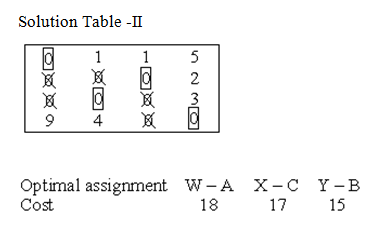Minimum cost = 18 + 17 + 15 = Rs 50